# Order of Operations Practice for Grade 3 Students

## What is Order of Operations?

Simply put, the “order of operations” in math is the sequence in which we solve problems with multiple operations. The “operations” are addition, subtraction, multiplication and division, among others. The “order” of these operations states which operation is calculated first, which operations is calculated second, and so on.

## So what is the order?

First, we solve the operation with parentheses, or brackets. In math, the parentheses are used to group things together.

For example:

5 × (14 − 8) + 3 =

5 x 6 + 3 =

Then, we solve any multiplication or division before any addition or subtraction parts of the problem.

Continuing our example, we see there is multiplication in the problem:

5 x 6 + 3 =

30 + 3 =

Finally, we perform the addition and subtraction.

In our example, there is addition involved:

30 + 3 = 33

## Order of operations worksheets for grade 3 students

In our grade 3 math section, we introduce order of operations where students add, subtract and multiply expressions with multiple operations. Division is introduced in our grade 4 order of operations worksheets.

## Order of operations with three numbers

In these worksheets, students practice adding and subtracting with a parenthesis.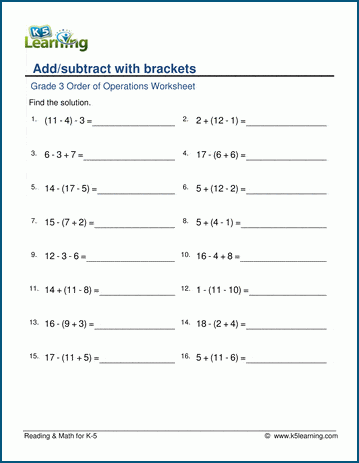## Order of operations with four numbers

The next level of worksheets has students working on 4 numbers in order of operations.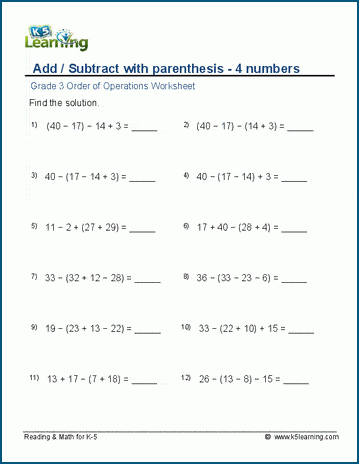## Order of operations with five numbers

A little more difficult, these worksheets deal with expressions of five numbers in multiple operations.## Order of operations with six numbers

In these most difficult worksheets for grade 3, students work with 6 terms.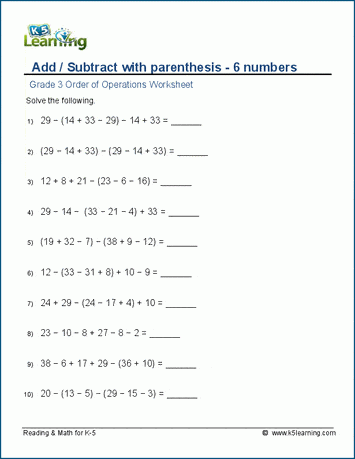## Adding, subtracting, and multiplying worksheets with no parenthesis

In these worksheets students with order of operations with no parenthesis.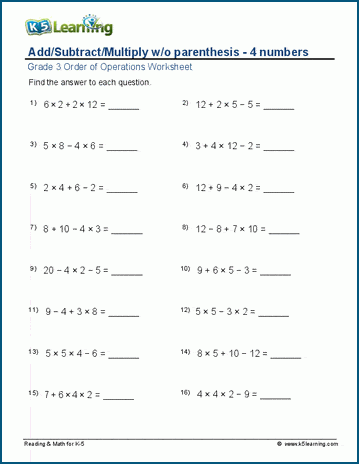## Adding, subtracting, and multiplying worksheets with parenthesis

Students add and subtract with parentheses in these worksheets.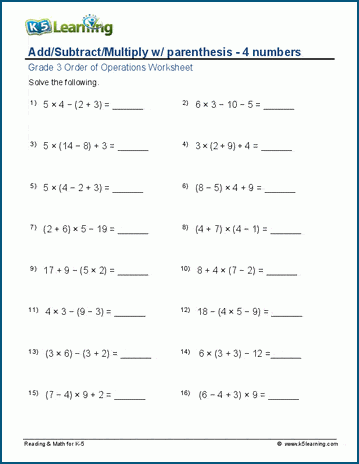## Adding, subtracting, and multiplying with parenthesis – five numbers

Students practice five terms in solving the order of operations equations.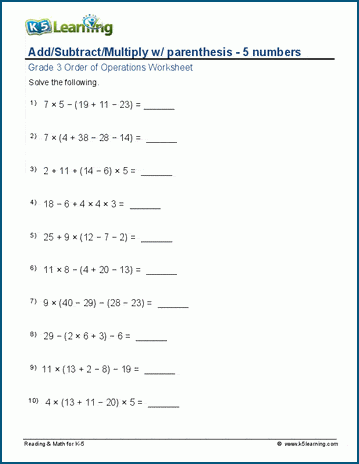## Adding, subtracting, and multiplying with parenthesis – six numbers

The last of these sets of worksheets have students work with six terms.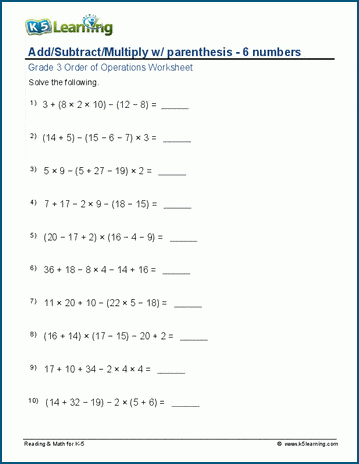Become a Member

This content is available to members only.